Home > A2C > Chapter 7 > Lesson 7.3.5 > Problem7-225

7-225.
1. Add or subtract and simplify each of the following expressions. Justify that each step of your process makes sense. Homework Help ✎

1.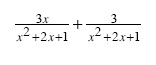2.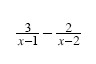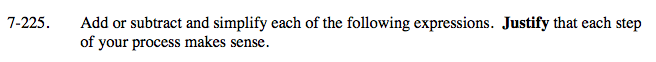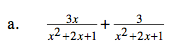Because the denominators are the same, simply add the numerators.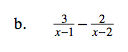To get a common denominator:

$\text{Multiply the first fraction by } \frac{x-1}{x-1}$

$\text{and the second fraction by } \frac{x-2}{x-2}.$

When subtracting, distribute the negative to all terms in the numerator of the second fraction.